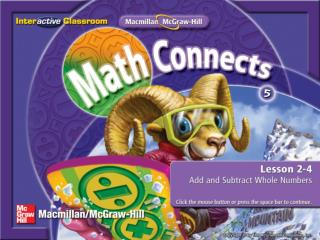Download PresentationSplash Screen

# Splash Screen

Download Presentation## Splash Screen

- - - - - - - - - - - - - - - - - - - - - - - - - - - E N D - - - - - - - - - - - - - - - - - - - - - - - - - - -
##### Presentation Transcript

1. Splash Screen

2. Five-Minute Check (over Lesson 2–3) Main Idea Example 1: Add or Subtract Whole Numbers Example 2: Add or Subtract Whole Numbers Example 3: Add with Regrouping Example 4: Subtract with Regrouping Lesson Menu

3. I will add and subtract multi-digit numbers. Main Idea/Vocabulary

4. Estimate321 + 608300 + 600 = 900 608 608 + 321 + 321 9 29 Add or Subtract Whole Numbers Find 321 + 608. Step 2 Add the tens. Step 3 Add the hundreds. Step 1 Add the ones. 608 + 321 9 2 9 CheckSince 929 is close to the estimate, the answer is reasonable. Example 1

5. A B C D Find 432 + 547. • 968 • 979 • 988 • 989 Example 1

6. Estimate3,879 – 535 3,900 – 500 = 3,400 3,879 3,879 – 535 – 535 4 44 Add or Subtract Whole Numbers Find 3,879 – 535. Step 2 Subtract the tens. Step 3 Subtract the hundreds and thousands. Step 1 Subtract the ones. 3,879 – 535 3, 3 4 4 CheckSince 3,344 is close to the estimate, the answer makes sense. Example 2

7. A B C D Find 4,788 – 254. • 4,534 • 4,634 • 4,644 • 4,645 Example 2

8. Add with Regrouping The high school band took a trip to Austin to see The Music Man. The music boosters raised \$6,253 for the trip, and students paid the remaining \$4,986. How much money did the trip cost in all? Estimate \$6,253 + \$4,986 → \$6,000 + \$5,000 = \$11,000 Example 3

9. 1 \$6,253 \$6,253 + \$4,986 + \$4,986 9 39 Add with Regrouping Step 2 Add the tens. Step 3 Add the hundreds. Add the thousands. Step 1 Add the ones. 1 1 \$6,253 Rename 13 tens as 1 hundred and 3 tens. + \$4,986 9 3 \$11, 2 Answer:The trip cost \$11,239 in all. The answer is close to the estimate. So, the solution is reasonable. Example 3

10. A B C D The school raised \$5,682 for the seniors to go on their senior trip. The students had to pay the remaining \$6,731. How much did the trip cost in all? • \$12,213 • \$12,412 • \$12,413 • \$13,413 Example 3

11. Estimate951 – 787 1,000 – 800 = 200 Subtract with Regrouping Find 951 – 787. Step 1 Subtract the ones. 11 4 951 1 < 7, so rename 5 tens as 4 tens, 10 ones. Then 10 ones + 1 one = 11 ones. – 787 4 Example 4

12. 11 11 4 4 951 951 – – 787 787 64 4 14 8 Subtract with Regrouping Step 2 Subtract the tens. 14 8 4 < 8, so rename 9 hundreds as 8 hundreds, 10 tens. Then 10 tens + 4 tens = 14 tens. 6 Step 3 Subtract the hundreds. 1 Answer:951 – 787 = 164 Example 4

13. A B C D Find 823 – 589. • 435 • 433 • 334 • 234 Example 4

14. End of the Lesson End Lesson

15. Five-Minute Check (over Lesson 2–3) Image Bank Math Tool Chest Add and Subtract Decimals Resources

16. A B C D (over Lesson 2–3) Solve. Use the work backward strategy. Marita’s dad made fruit bars for the bake sale. After selling 34, eating 2, and giving away 4, there were 8 fruit bars left over. How many fruit bars did Marita’s dad bake in all? • 50 fruit bars • 49 fruits bars • 48 fruit bars • 47 fruit bars Five Minute Check 1

17. End of Custom Shows